Courses
Courses for Kids
Free study material
Free LIVE classes
More

# Addition of Vectors - JEE Important TopicLIVE
Join Vedantu’s FREE Mastercalss

The addition of two or more vectors using the sum operator to obtain a new vector is known as vector addition. This new vector is obtained by summing up the other vectors (be it two or more than two vectors). There are different laws that help with the addition of vectors like parallelogram law, triangle law, and polygon law. Displacement, velocity, and acceleration all are vectors, and thus, vector addition is frequently used for these quantities. Let us understand in-depth what is vector addition and some of its properties.

## What is the Addition of Vectors in Cartesian Form?

In cartesian form, a 2D vector in the vector plane is represented as $\vec{A}=x \hat{i}+y \hat{j}$ and a 3D vector is represented as $\vec{A}=x \hat{i}+y \hat{j}+z \hat{k}$. A 3D vector is as represented below. Here, Ax represents the component of vector A along the x-axis, Ay represents the component of vector A along the y-axis and Az represents the component of the vector along the z axis.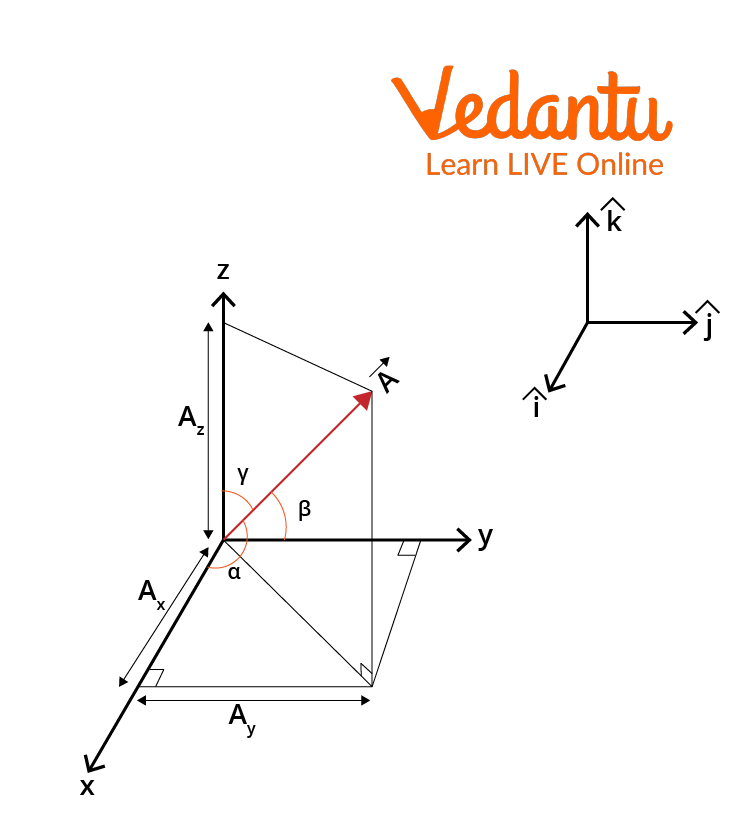3D Vector in Cartesian Form

To add the vectors in cartesian form, let us consider two vectors $\vec{A}$ and $\vec{B}$. Assuming the vectors to be 2D in cartesian form they will be denoted as:

$\vec{A}=A_{x} \hat{i}+A_{y} \hat{j} \text { and } \vec{B}=B_{x} \hat{i}+B_{y} \hat{j}$

To find the resultant for the above two vectors we add the vectors in this way:

$\vec{A}+\vec{B}=\left(A_{x}+B_{x}\right) \hat{i}+\left(A_{y}+B_{y}\right) \hat{j}$

where $\hat{i}$ and $\hat{j}$ denote directions.

Let us understand how this can be achieved.Resultant R of Two Vectors

It can be seen from the figure above that

\begin{align} &R_{x}=A_{x}+B_{x} \\ &R_{y}=A_{y}+B_{y} \\ &\vec{R}=R_{x} \hat{i}+R_{y} \hat{j} \\ &\vec{R}=\left(A_{x}+B_{x}\right) \hat{i}+\left(A_{y}+B_{y}\right) \hat{j} \\ &\vec{A}+\vec{B}=\left(A_{x}+B_{x}\right) \hat{i}+\left(A_{y}+B_{y}\right) \hat{j} \end{align}

This, proves that the two vectors in cartesian form can be added in the form $\vec{A}+\vec{B}=\left(A_{x}+B_{x}\right) \hat{i}+\left(A_{y}+B_{y}\right) \hat{j}$

## State Different Laws for Vector Addition

A vector $\overrightarrow{X Y}$ simply means the displacement from point X to point Y. Now imagine a person walks from a point X to Y and then moves from point Y to Z. Thus, the net displacement of the person from point X to Z is represented by $\overrightarrow{X Y}$

Mathematically, the above scenario can be represented as $\overrightarrow{X Z}=\overrightarrow{X Y}+\overrightarrow{Y Z}$. This is known as the triangle law of vector addition. Let us further understand what is the triangle law of vector addition.

Suppose there are two vectors $\vec u$ and $\vec v$, wherein the initial point of one vector coincides with the terminal point of another vector. Then the vector addition in this case will be the vector sum of the two vectors $\vec u$ and $\vec v$ i.e. $\vec{u}+\vec{v}$. The vectors that form the three sides of the triangle when added in order to measure $\vec 0$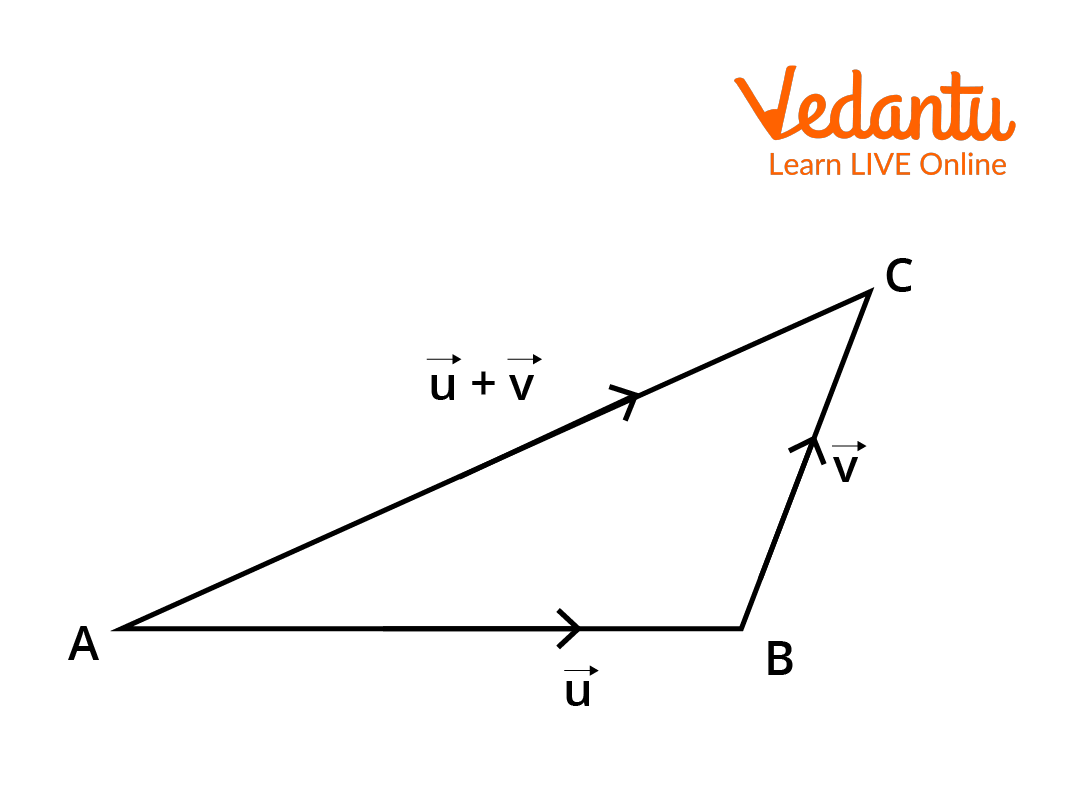Pictorial Representation of the Triangle Law of Vector Addition

Now, the vector law of addition can be further classified into:

1. Parallelogram Law and

2. Polygon Law

1. Parallelogram Law of Vector Addition

The triangle law of addition and parallelogram law of vector addition are equivalent to one another. Let us further understand this with an example.

Imagine a sailboat is moving in a river from one bank to another. The wind direction is making the sailboat move in a direction perpendicular to the flow of the river. It is understandable that there are two velocities involved, one is the velocity imparted by the wind ($\vec P$) which is moving the sailboat and the other velocity is due to the flow of a river ($\vec Q$). Under the influence of the two velocities on the sailboat, it starts moving with a different velocity ($\vec R$) which is also the resultant velocity.

If  $\vec P$ and $\vec Q$ are the two adjacent sides of a parallelogram as shown in the figure below then $\vec R$ represents the diagonal of the parallelogram. This is known as the parallelogram law of vector addition.

Mathematically, this can be denoted as $\overrightarrow{O A}+\overrightarrow{O B}=\overrightarrow{O C}$ or $\vec{P}+\vec{Q}=\vec{R}$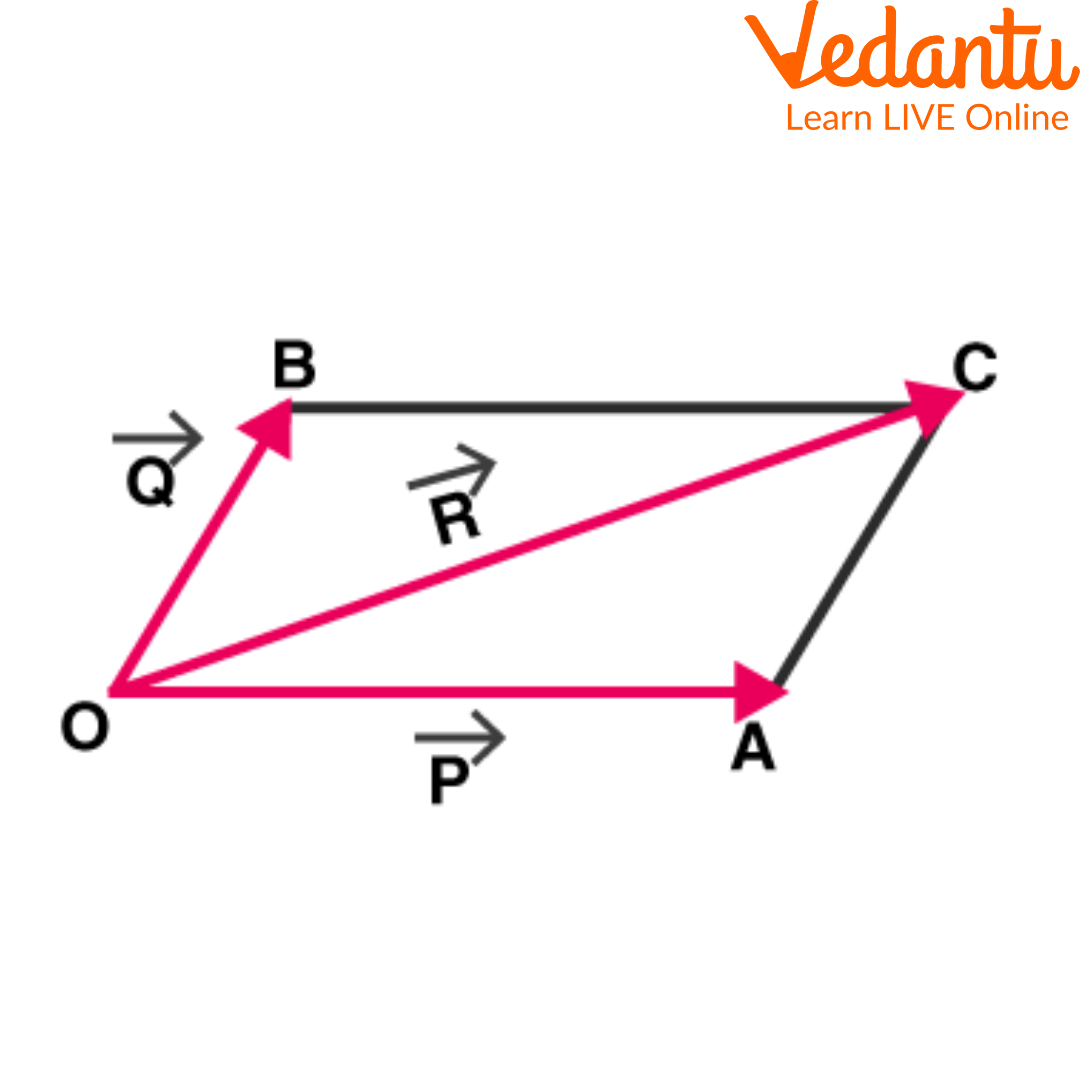Consider Another Parallelogram as Shown in the Figure below: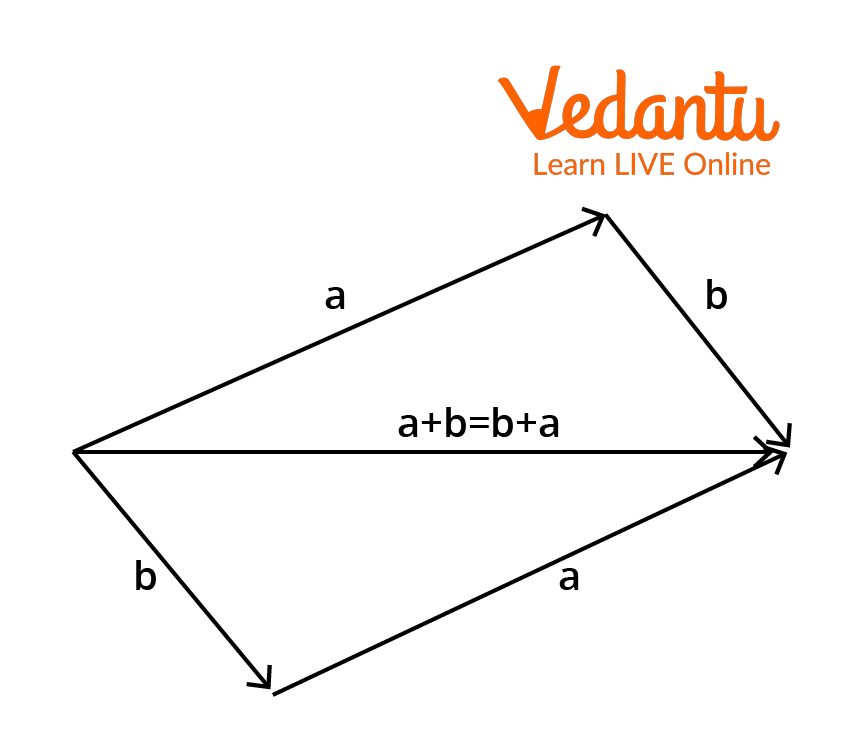Here, the diagonal of the parallelogram is represented by $\vec{a}+\vec{b}$ by using the triangle law of vector addition. We know that the opposite sides of a parallelogram are equal and parallel. Thus, the opposite sides will have the same magnitude and again the diagonal of the parallelogram measures $\vec{b}+\vec{a}$. Thus, the diagonal of the parallelogram can be defined as $\vec{a}+\vec{b}$ or $\vec{b}+\vec{a}$.

2. Polygon Law of Vector Addition

If a number of vectors can be represented in magnitude and direction by the sides of a polygon taken in the same order, then their resultant may be represented in magnitude and direction by the closing side of the polygon taken in the opposite order, according to the polygon law of vector addition.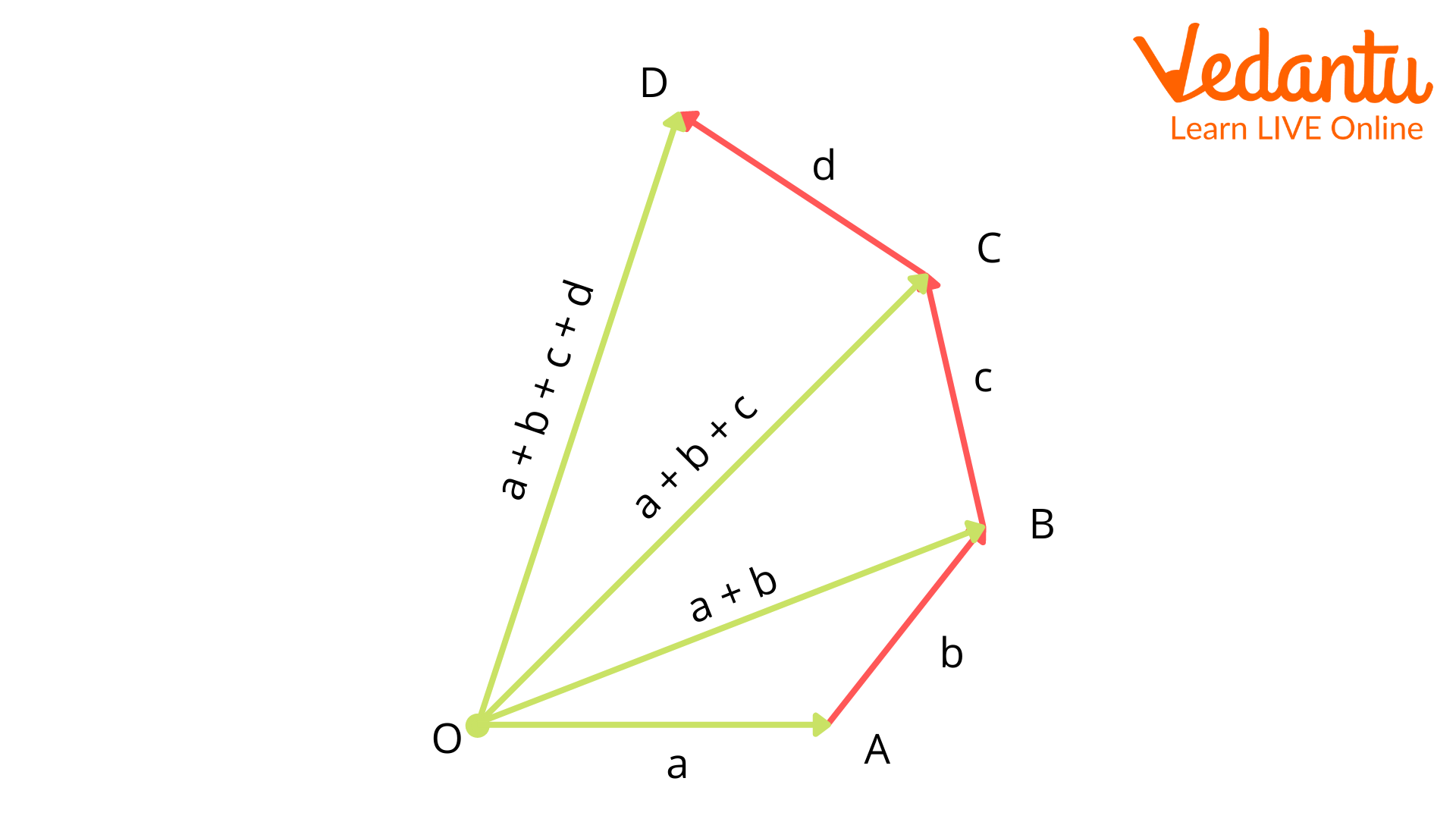## How to Add Vectors Graphically?

It is evident from above that vectors can be added graphically using the triangle law (or head-to-tail rule), the parallelogram method, and using the components. The third method using the components is the same as vector addition in cartesian form. If the components of a vector are given we can determine the resultant vector as discussed in an earlier section.

## What are the Properties of Vector Addition?

Vector addition entails different properties. The three most important properties of vector addition are listed below:

1. Commutative Property

2. Associative Property

1. Commutative Identity

The commutative identity is given as for any two arbitrary vectors a and b,

a+ b = b + a

2. Associative Property

The associative poetry is given as for any three arbitrary vectors p, q, and r as p + q + r = r + q + p, which means that the order in which we add the vectors does not matter.

The additive identity is given as a + 0 = 0 + a, where a is any arbitrary vector and 0 is the zero vector. Here, O represents the additive identity.

## Solved Examples on Properties of Vector Addition

1. Find the resultant of vectors $\vec{A}=2 \hat{i}+3 \hat{j}+2 \hat{k}$ and $\vec{B}=3 \hat{i}+6 \hat{j}+2 \hat{k}$ using the commutative identity.

Solution: From above, we know that the commutative identity, $\vec{A}+\vec{B}=\vec{B}+\vec{A}$

\begin{align} &\vec{A}+\vec{B}=(2 \hat{i}+3 \hat{j}+2 \hat{k})+(3 \hat{i}+6 \hat{j}+2 \hat{k})=(5 \hat{i}+9 \hat{j}+4 \widehat{k}) \\ &\vec{B}+\vec{A}=(3 \hat{i}+6 \hat{j}+2 \widehat{k})+(2 \hat{i}+3 \hat{j}+2 \hat{k})=(5 \hat{i}+9 \hat{j}+4 \hat{k}) \end{align}

Therefore, $\vec{A}+\vec{B}=\vec{B}+\vec{A}$

2. Find the resultant of vectors $\vec{A}=2 \hat{i}+3 \hat{j}+2 \hat{k}$,  $\vec{B}=3 \hat{i}+6 \hat{j}+2 \hat{k}$, and $C=\hat{i}+2 \hat{j}+4 \hat{k}$ using the associative identity.

Solution: From above, we know the associative identity is $\vec{A}+\vec{B}+\vec{C}=\vec{C}+\vec{A}+\vec{B}$

$\begin{array}{r} \vec{A}+\vec{B}+\vec{C}=(2 \hat{i}+3 \hat{j}+2 \hat{k})+(3 \hat{i}+6 \hat{j}+2 \hat{k})+(1 \hat{i}+2 \hat{j}+4 \hat{k})=6 \hat{i}+11 \hat{j}+8 \hat{k} \\ \vec{A}+\vec{B}+\vec{C}=\vec{C}+\vec{A}+\vec{B}=(1 \hat{i}+2 \hat{j}+4 \hat{k})+(2 \hat{i}+3 \hat{j}+2 \hat{k})+(3 \hat{i}+6 \hat{j}+2 \hat{k})=6 \hat{i}+11 \hat{j}+8 \hat{k} \end{array}$

Therefore, $\vec{A}+\vec{B}+\vec{C}=\vec{C}+\vec{A}+\vec{B}$

## Conclusion

The article thoroughly summarises the vector addition by using different examples. A walkthrough of the different laws of vector addition have also been discussed. Different properties of vector addition have also been discussed that make the calculation of vector addition simpler. Also, it must be noted that two or more vectors can only be added if they have the same dimensions and units.  Vectors are employed in several fields, and so does the vector addition. Some of these fields include quantum mechanics, engineering, and Mathematics.

Last updated date: 24th Sep 2023
Total views: 131.7k
Views today: 4.31k

## FAQs on Addition of Vectors - JEE Important Topic

1. What are the different types of vectors?

The different types of vectors are zero vector, unit vector, coinitial vectors, collinear, and equal vectors. The zero vector is also called the null vector. The null vector has a zero magnitude. Also, it can be regarded to have any direction. The vector which has magnitude one is called the unity or unit vector. The direction of this vector is in the direction of a given vector $\vec u$. The vectors with the same initial point  and the vectors that are parallel to the same line are called coinitial and collinear vectors, respectively. Equal vectors are the vectors that have the same magnitude and direction, i.e., $\vec x=\vec y$.

2. What is the negative of a vector?

A vector that has the same magnitude as that of a given vector but its direction is opposite to it is called the negative of a vector. For example, the vector $\vec {QR}$ is the negative of vector $\vec {RQ}$. Thus, the negative vector can be represented as $\vec {QR}=-\vec {RQ}$. The minus (-) sign here indicates the direction of a vector opposite to that of a reference vector. A car travelling 10 miles north and another travelling 10 miles south can be denoted as two vectors $\vec A$ and $\vec B$, respectively. The two are travelling in opposite directions, thus can be denoted as $\vec A=-\vec B$. Here, $\vec B$ is the negative of vector $\vec A$.